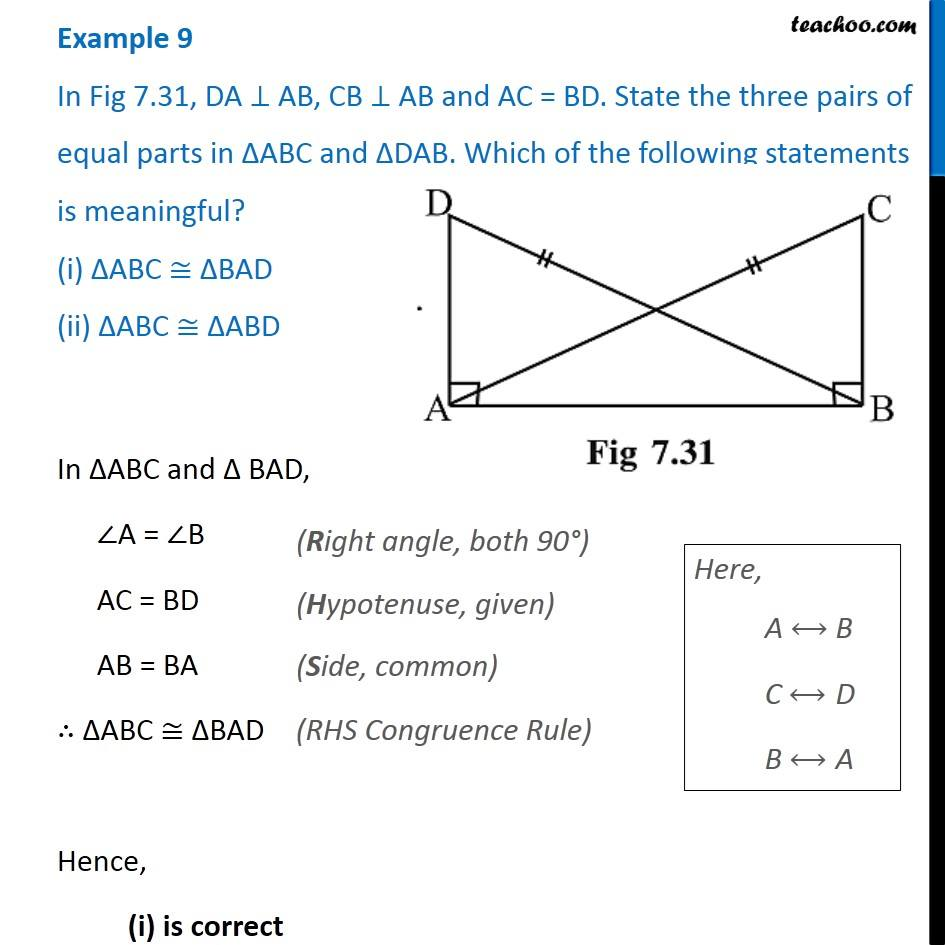Examples

Chapter 7 Class 7 Congruence of Triangles
Serial order wise∠A = ∠B

AC = BD

AB = BA

Hence,

( i ) is correct

### Transcript

Example 9 In Fig 7.31, DA ⊥ AB, CB ⊥ AB and AC = BD. State the three pairs of equal parts in ∆ABC and ∆DAB. Which of the following statements is meaningful? (i) ∆ABC ≅ ∆BAD (ii) ∆ABC ≅ ∆ABD In ∆ABC and ∆ BAD, ∠A = ∠B AC = BD AB = BA ∴ ∆ABC ≅ ∆BAD Hence, (i) is correct